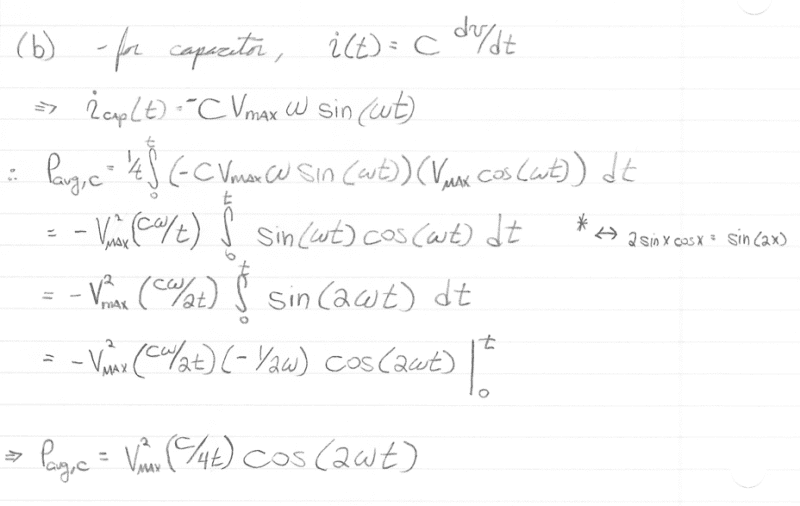# Circuits: Average Power Absorbed by Elements

## Homework Statement

Let $v(t) = V_{max}\cos(\omega t )$ be applied to (a) a pure resistor, (b) a pure capacitor (with zero initial capacitor voltage) and (c) a pure inductor (with zero initial inductor voltage). Find the average power absorbed by each element.

## Homework Equations

$$P_{avg}(\tau) = \frac{1}{t}\int_0^t i(\tau)v(\tau)\,d\tau$$

Ohms law.

## The Attempt at a Solution

Please skip ahead to the capacitor and inductor part of problem.

Resistor

combining Ohm's Law and the average power equation, we have

$$P_{avg,R} = \frac{1}{t}\int_0^t \frac{v(\tau)}{R}v(\tau)\,d\tau$$

$$= \frac{1}{Rt}\int_0^t \left [V_{max}\cos(\omega \tau )\right ] ^2 \,d\tau$$

Using the trig identity: $2\cos(x)\cos(y) = \cos(x - y) + \cos(x+y)$

$$P_{avg,R} = \frac{V_{max}^2}{Rt}\int_0^t \cos(\omega \tau )\cos(\omega \tau )d\tau$$

$$= \frac{V_{max}^2}{2Rt}\int_0^t \left \{1 - \cos(2\omega\tau)\right \} \,d\tau$$

$$\Rightarrow P_{avg,R} = \frac{V_{max}^2}{2Rt}\left \{\tau - \frac{1}{2\omega}\sin(2\omega\tau)\right \}_0^t$$

Hmmm ... I guess this does work. I thought that the units weren't going to check out, but I forgot that 1/omega gets me my time dimension back. Oh well. I guess I answered this part ok.

For the capacitor and inductor I am assuming I will go through a similar procedure, however, my text book makes a claim in the chapter that I disagree with. It says that the average power of a capacitor and inductor whose voltage and current vary sinusoidally can be shown to be zero. Is this ALWAYS true? Or only when the time interval of interest is some multiple of the period?

Last edited:

## Answers and Replies

Carrying out the procedure for a capacitor, I have the following:It seems to me that this is only zero when the t = t0 + nT where T is the period of sin(2wt) and n = 1,2,3 ...

Any thoughts? Is this correct that the average power is only zero over multiples of T or am I missing something?

Thanks!

Phrak
The text statement is incorrect.

The power certainly varies over time, which is why the exercise in finding average power is useful in the first place.

The instantaneous power is P(t)=i(t)v(t). For sinusoidal voltage it's evident that the power is not constant.

In order that the integral be invariant over any interval the integrand must be constant.

Last edited: